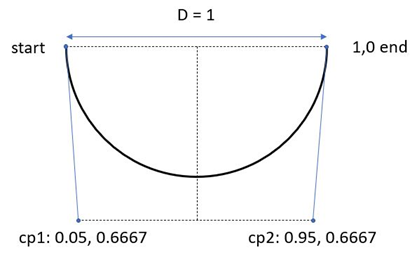Altair® Panopticon

#### Drawing a Circle with Cubic Bézier Curves

It is not possible to create a perfect circle with cubic Bézier curve commands, i.e., the c/C and s/S commands. A simple to use approximation of a circle that is created with just two Bézier curves is as follows:

The c command takes 3 points (x, y) as arguments: the first two are control points and the third is the end point. To draw the lower half of a circle with a diameter of 1, drawing from left to right, you can use these control point values. All points are expressed relative to the starting position. Remember that the y-axis is positive in the downwards direction.

controlpoint 1: x = 0.05, y = 0.6667

controlpoint 2: x = 0.95, y = 0.6667

end point: x = 1, y = 0

which makes:

c 0.05, 0.6667 0.95, 0.6667 1, 0 zTo draw a complete circle with a diameter of 1, you continue the c command with 3 more points, giving the two control points and the end point of the upper half of the circle. Note that you don't need to repeat the c command:

c 0.05, 0.6667 0.95, 0.6667 1, 0 -0.05, -0.6667 -0.95, -0.6667, -1, 0 z

So, the x-value of the first control point is 5% of the diameter, and the x-value of the second control point is 95% of the diameter.

The y-values are 2/3:s of the diameter. The sign of the relative point depends on the direction in which you are moving. Positive y-values are downwards.